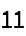Function Repository Resource:

SymbolExamples

Create a notebook of all documentation examples for a symbol

Contributed by: Michael Sollami
 ResourceFunction["SymbolExamples"][symbol] creates a notebook with the documentation page examples for symbol.

Details and Options

ResourceFunction["SymbolExamples"] relies on using EntityValue, which requires internet connectivity.
ResourceFunction["SymbolExamples"] is not yet supported in the Wolfram Cloud (since it relies on documentation URLs).

Examples

Basic Examples

View a notebook containing all the examples from the documentation page for Prime:

 In:=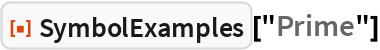Out=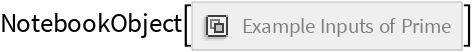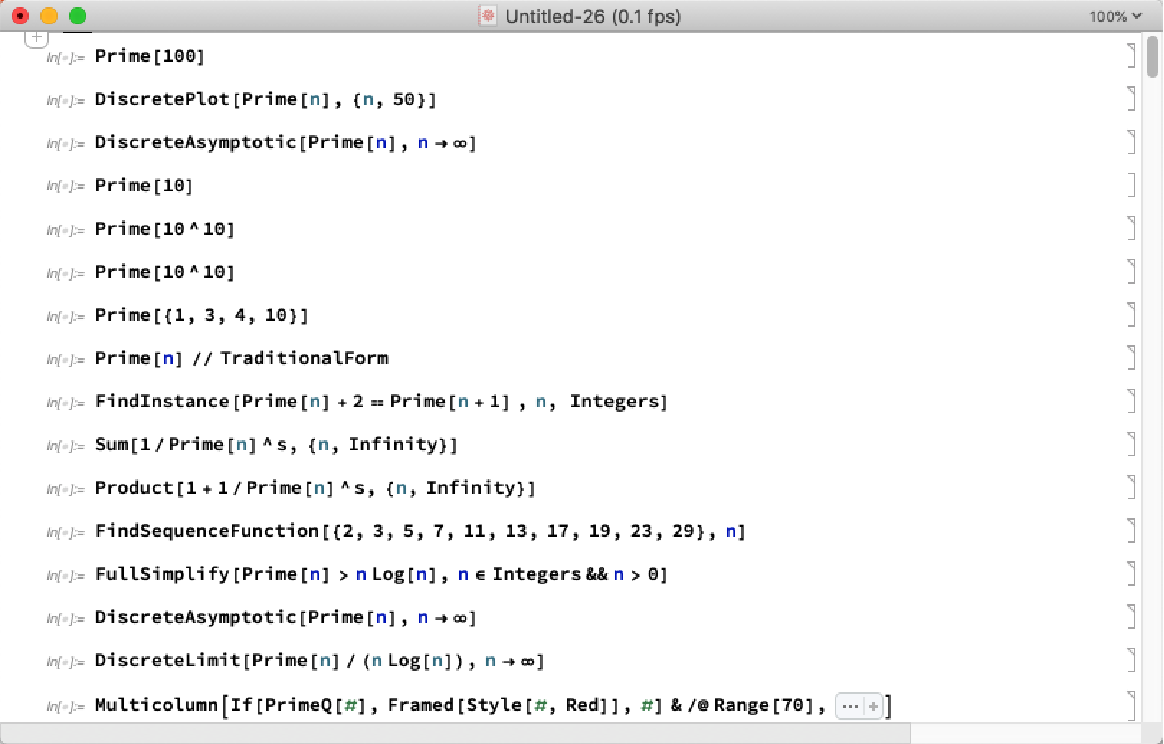Show all the examples of MeijerG:

 In:=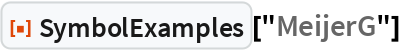Out=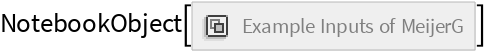Properties and Relations

SymbolExamples gives all examples, not just a sampling:

 In:=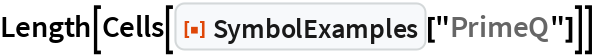Out=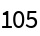Compare to WolframLanguageData, which gives a sample:

 In:=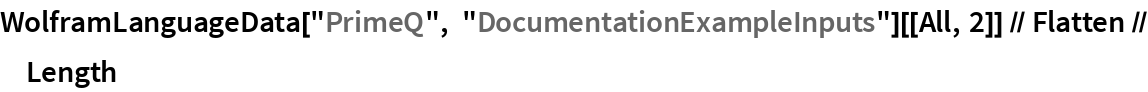Out=